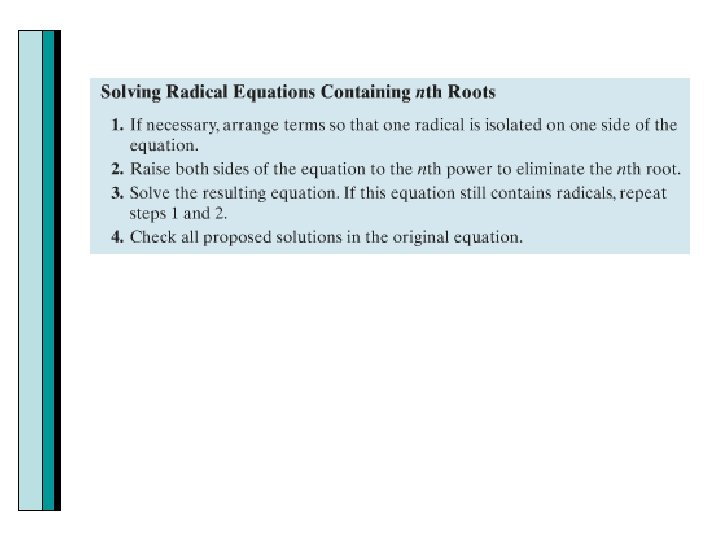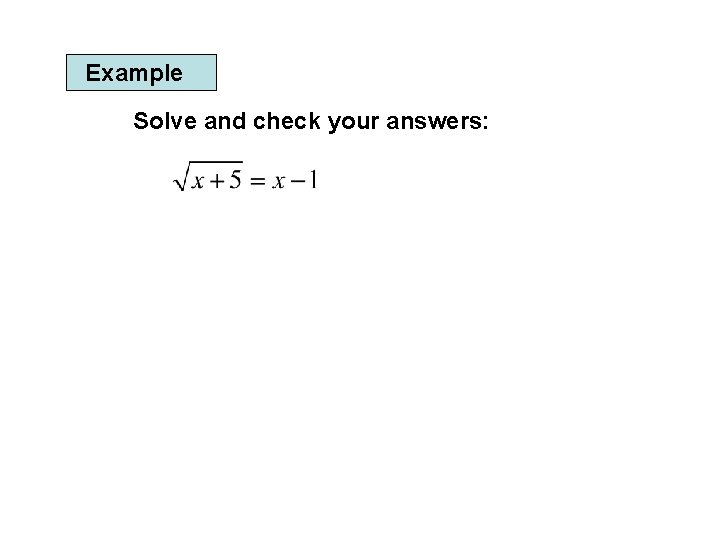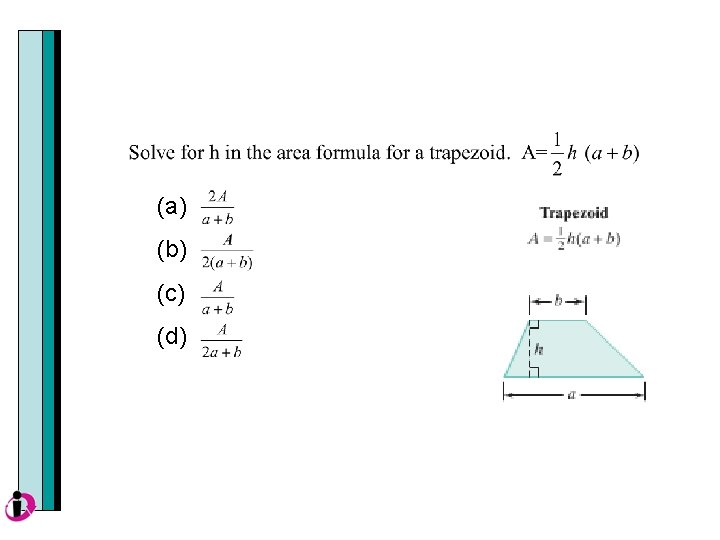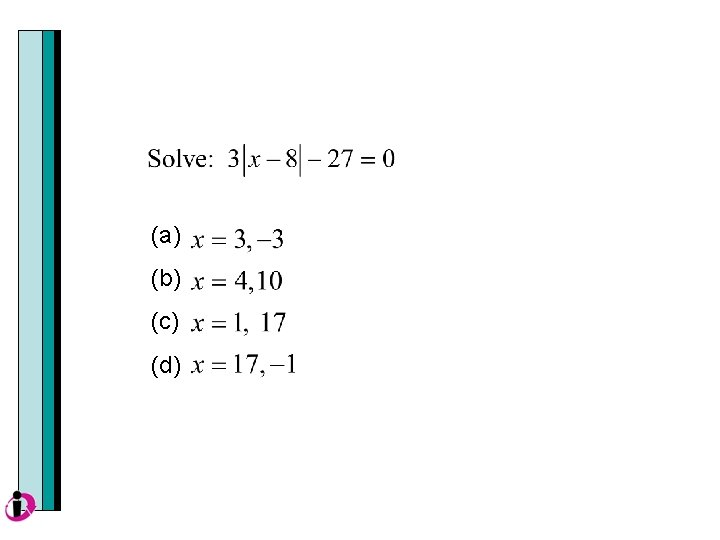# Section P 7 Equations Solving Linear Equations in

• Slides: 65Section P 7 EquationsSolving Linear Equations in One Variable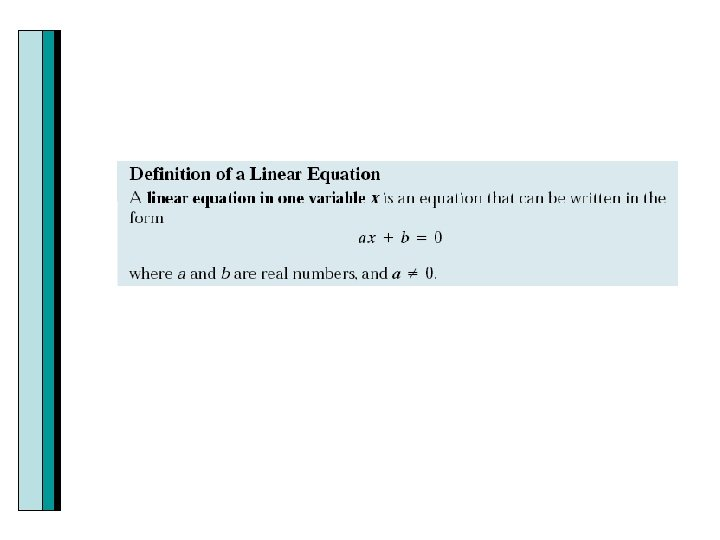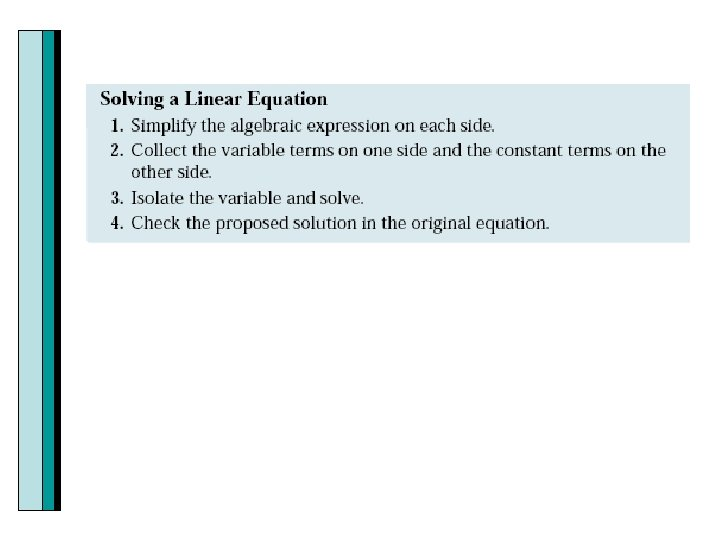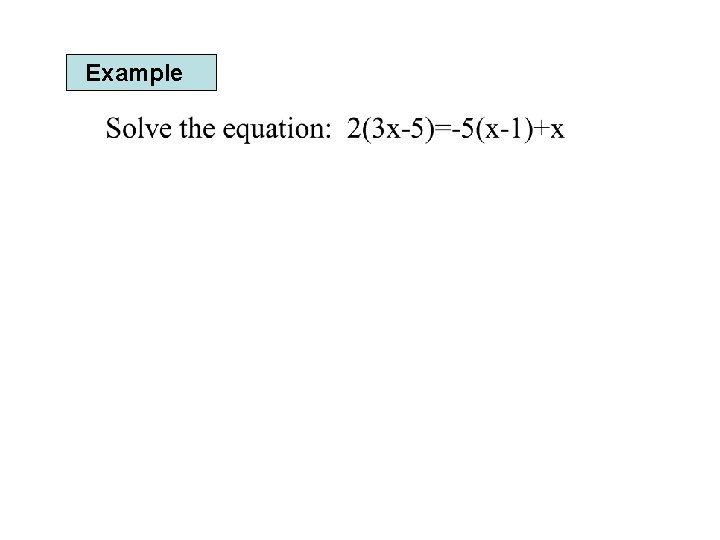ExampleLinear Equations with FractionsSolving with Fractions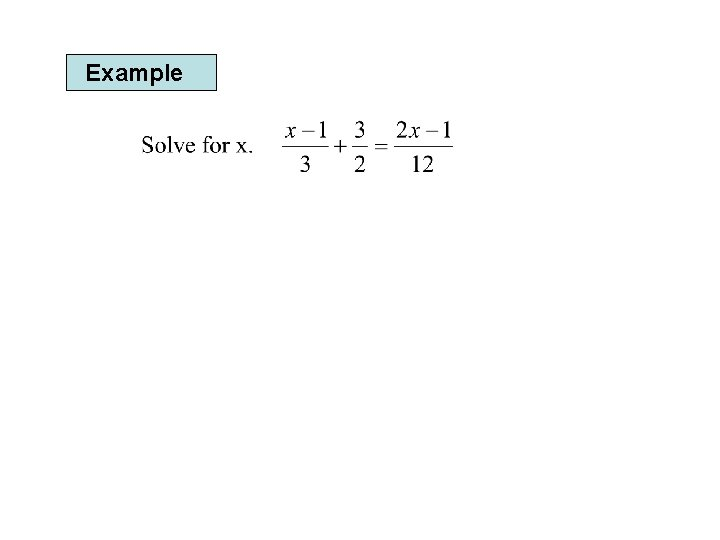Example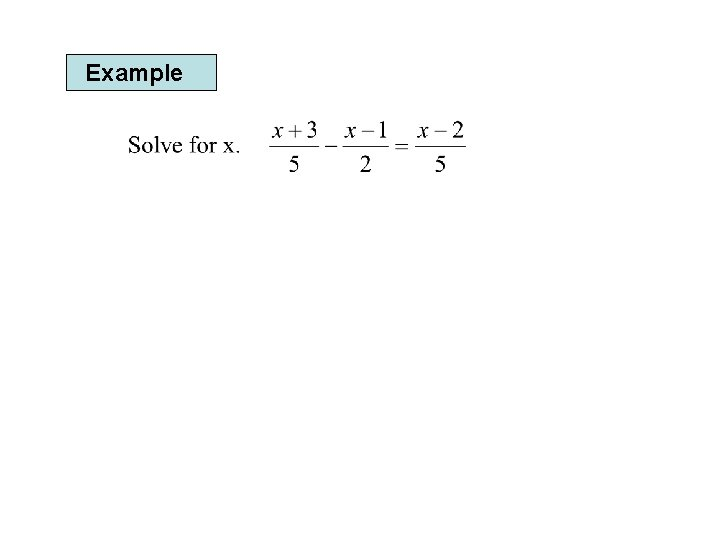ExampleRational Equations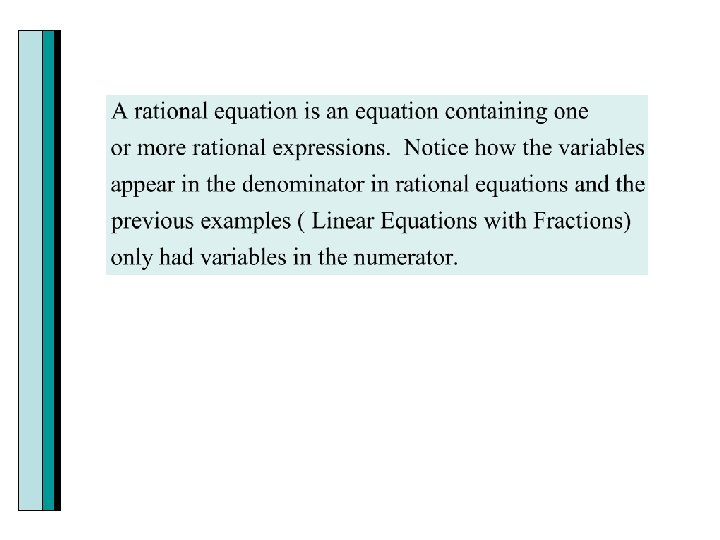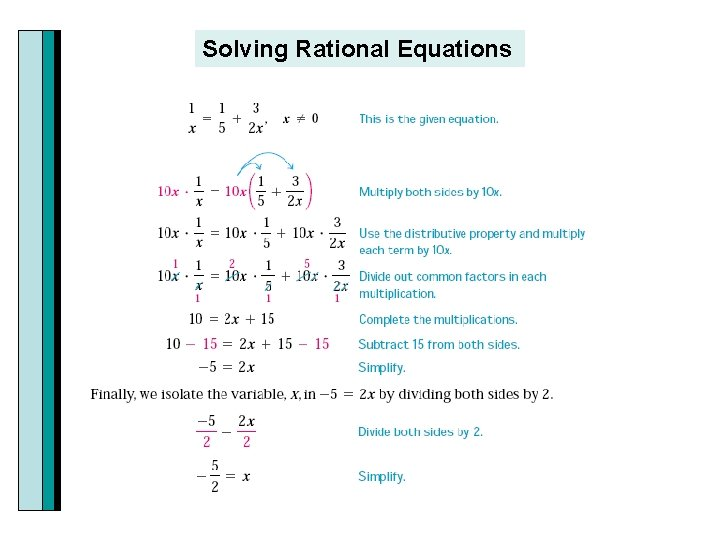Solving Rational Equations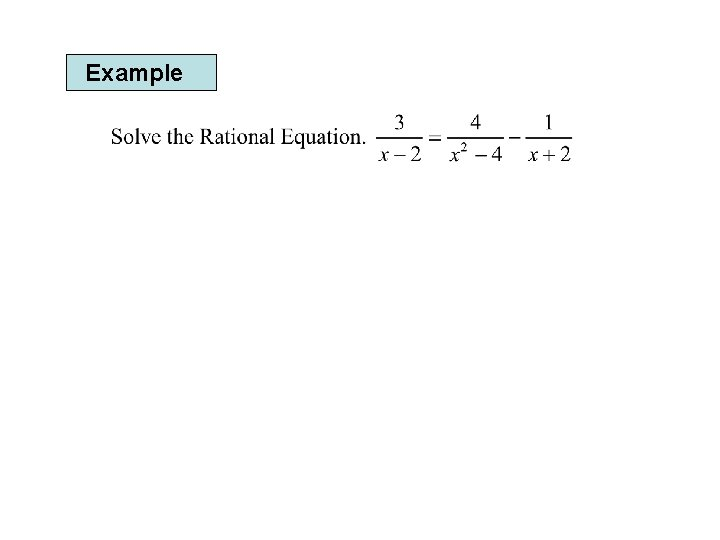Example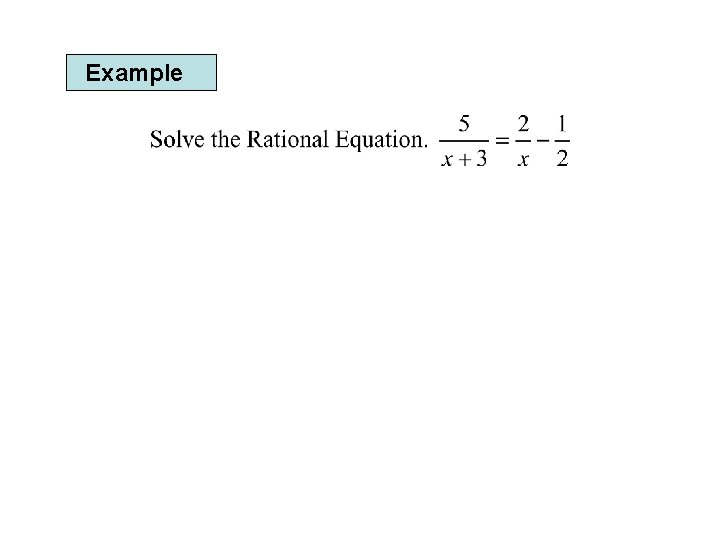ExampleExampleSolving a Formula for One of Its Variables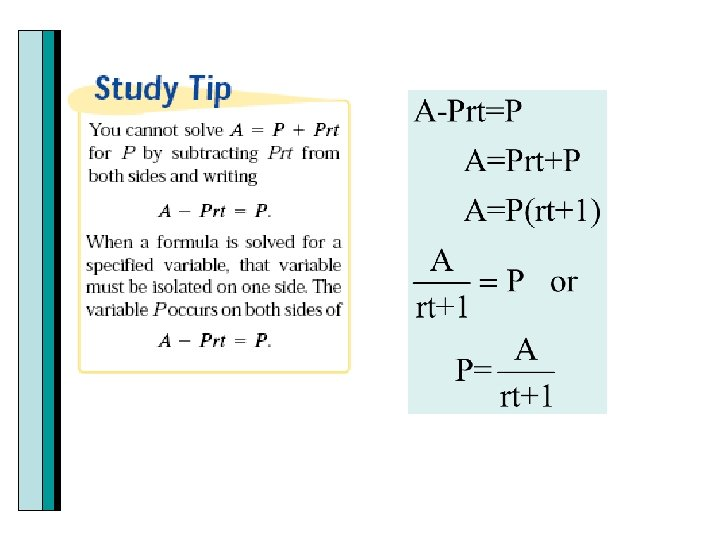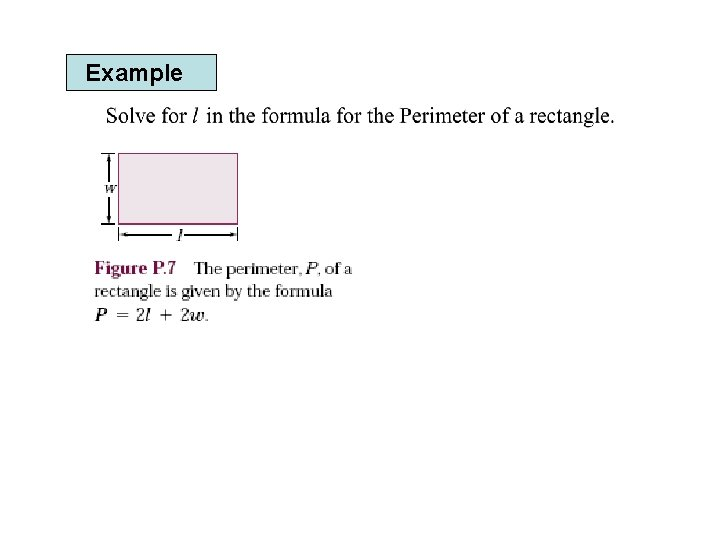Example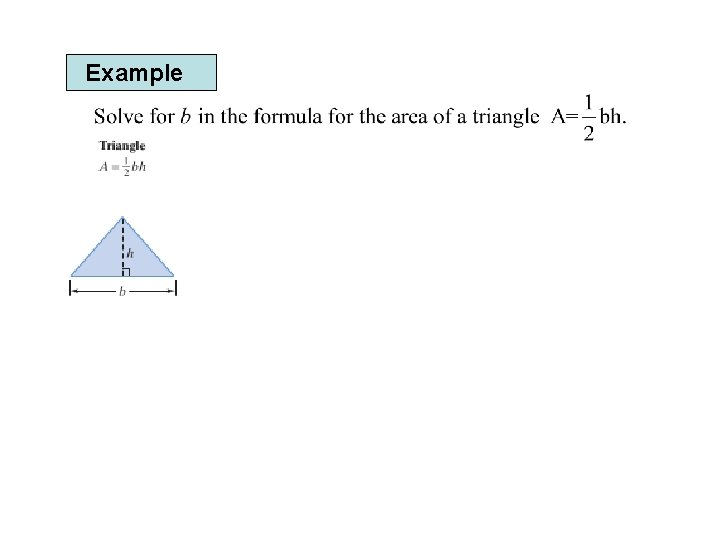ExampleEquations Involving Absolute Value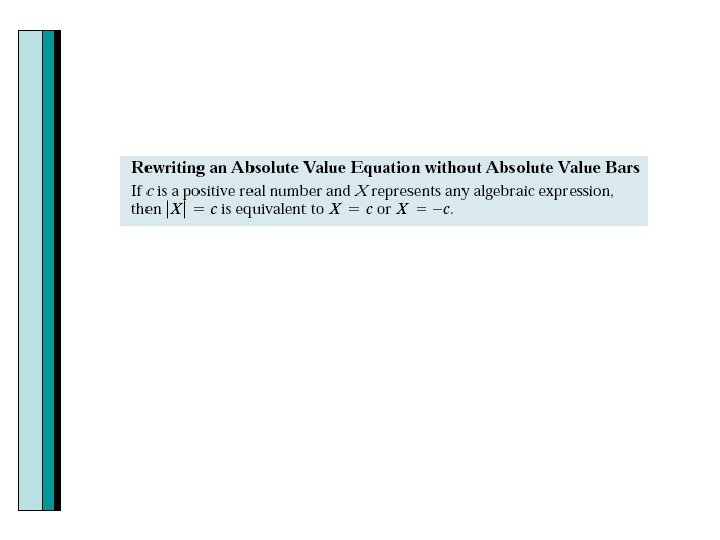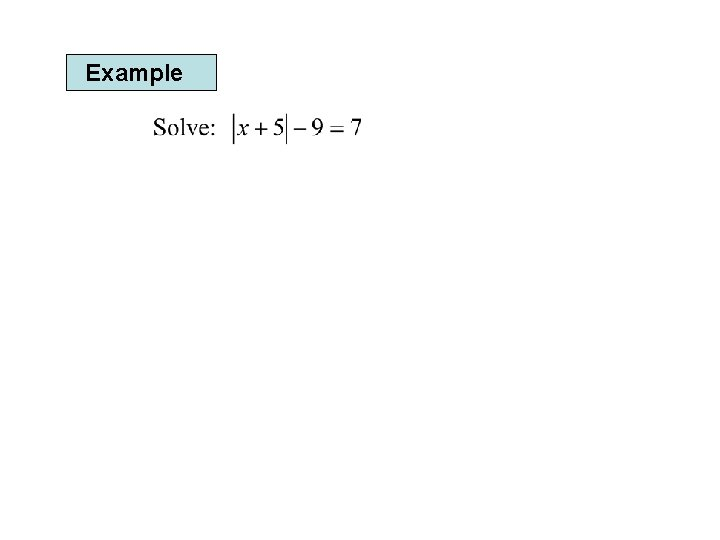ExampleExample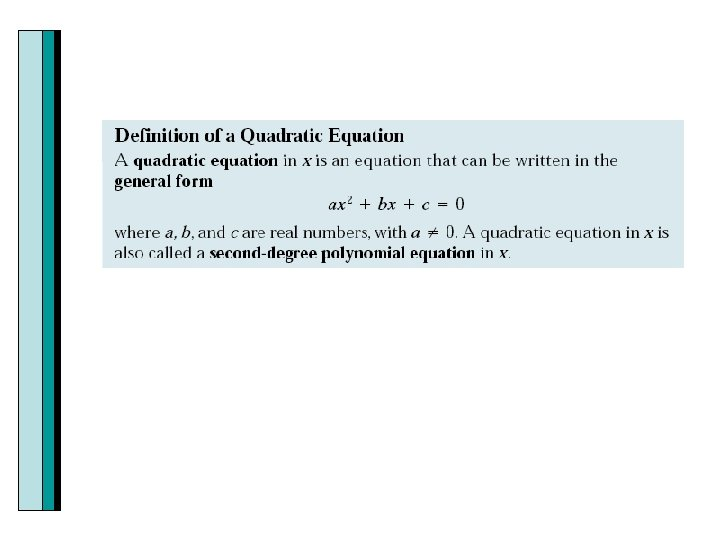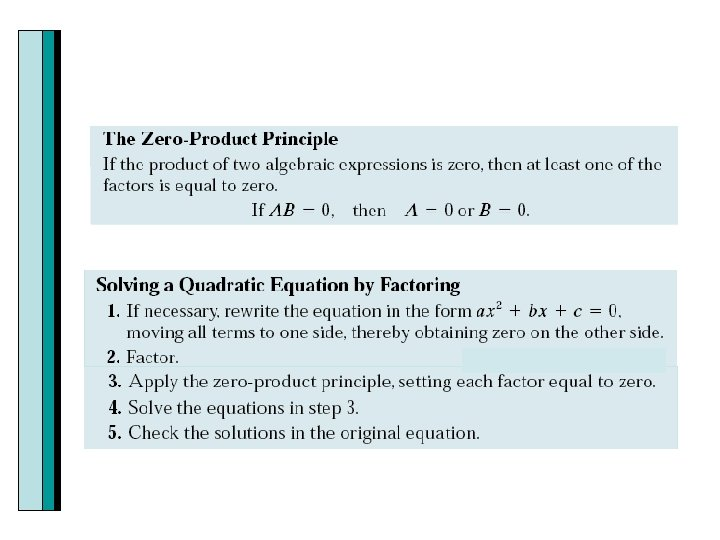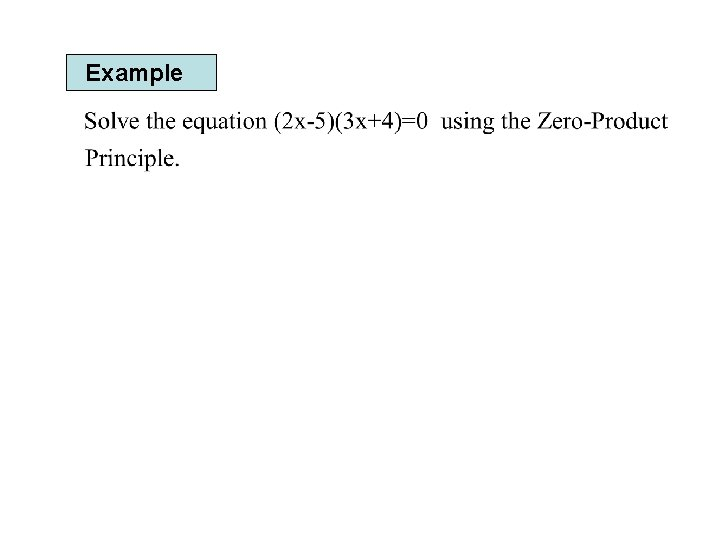Example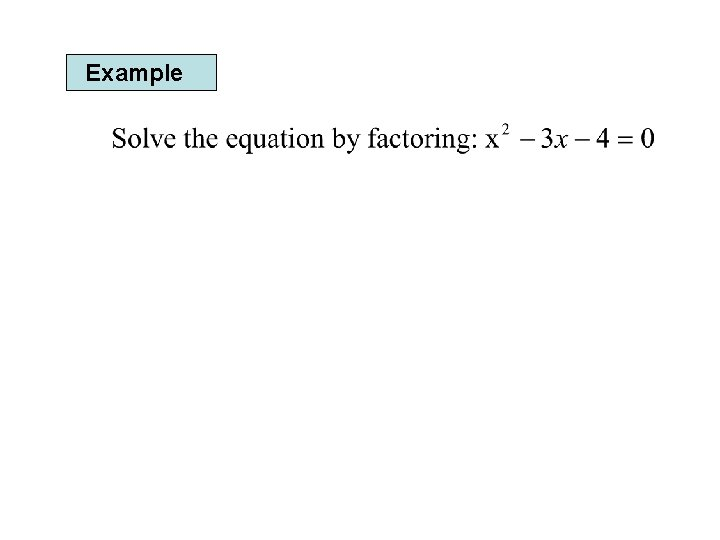Example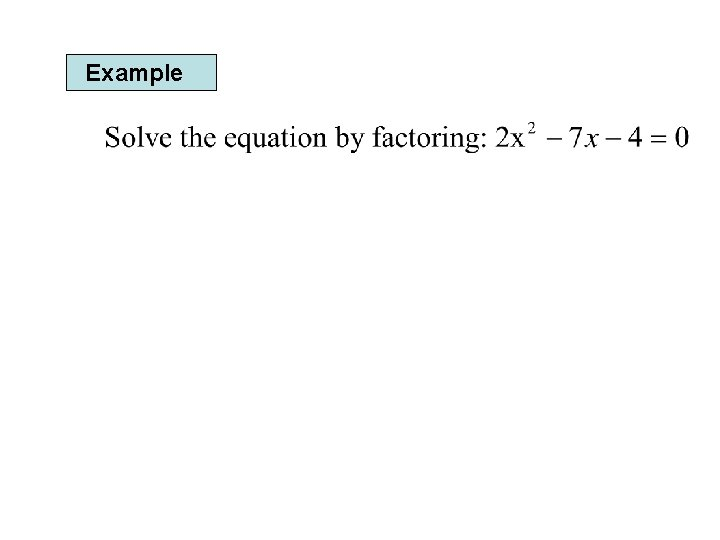Example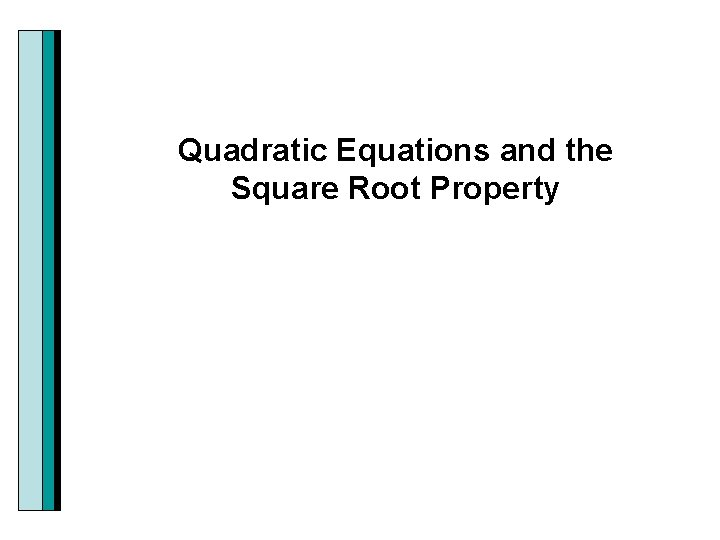Quadratic Equations and the Square Root Property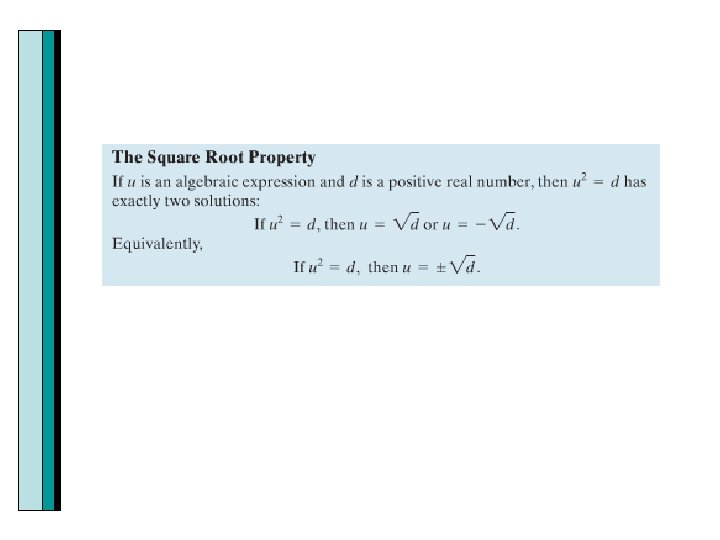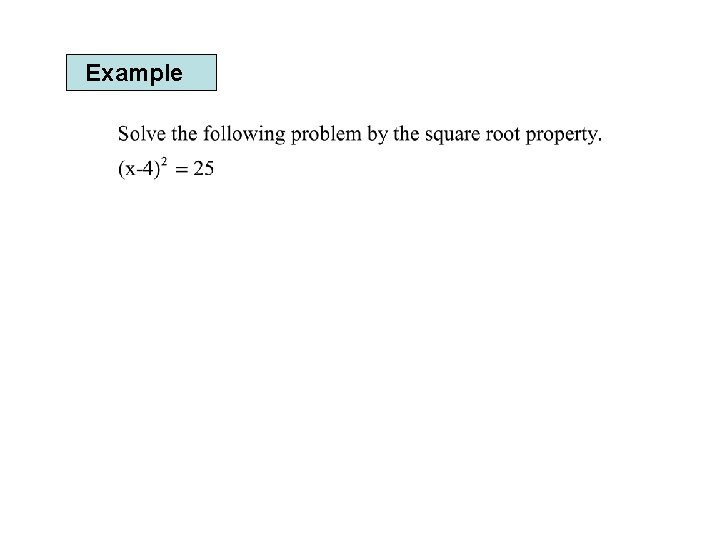ExampleExample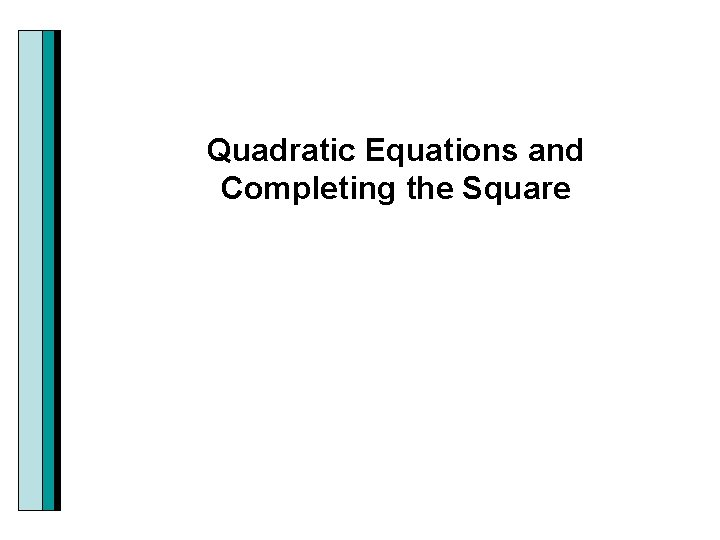Quadratic Equations and Completing the Square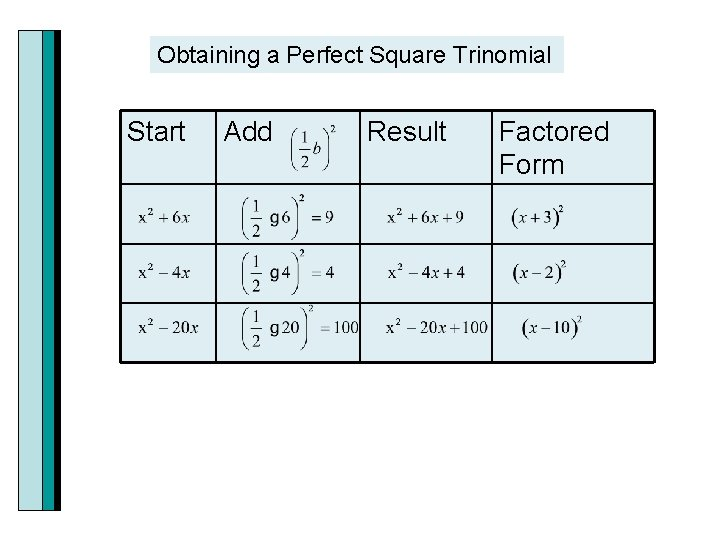Obtaining a Perfect Square Trinomial Start Add Result Factored Form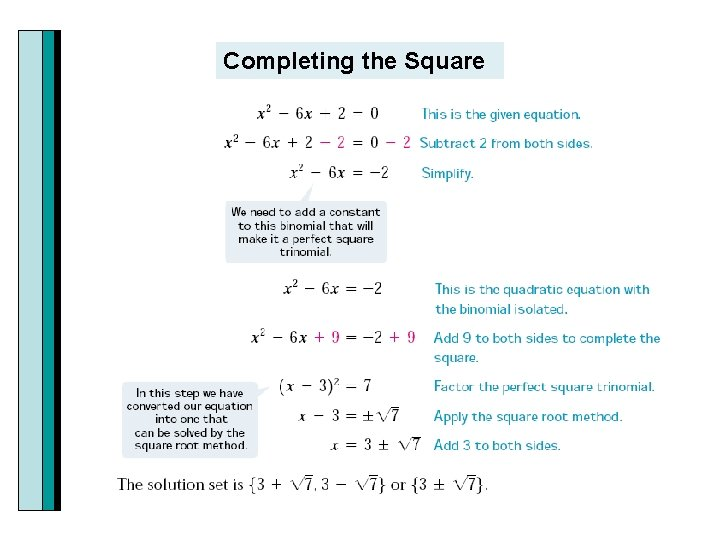Completing the Square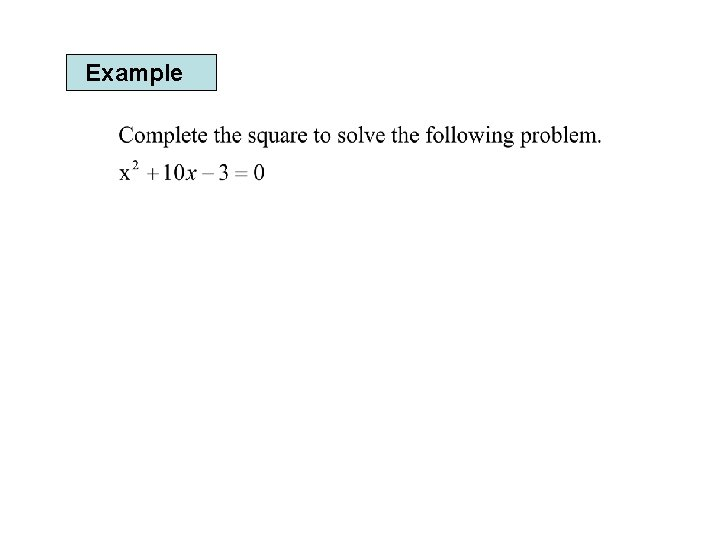ExampleExample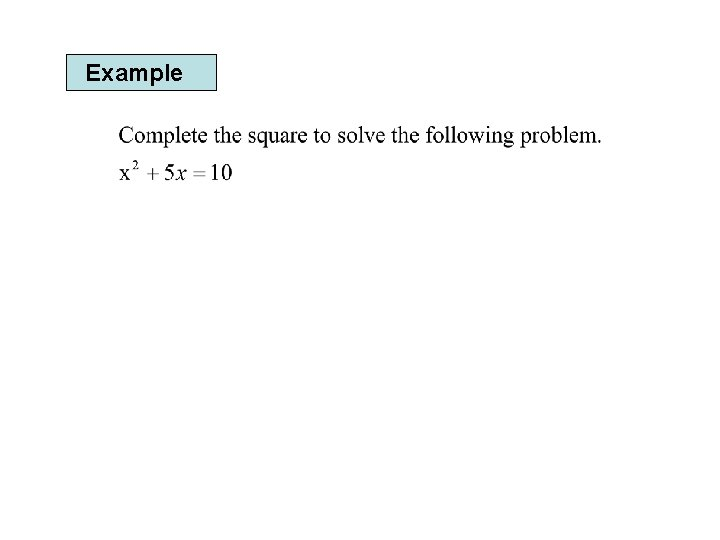Example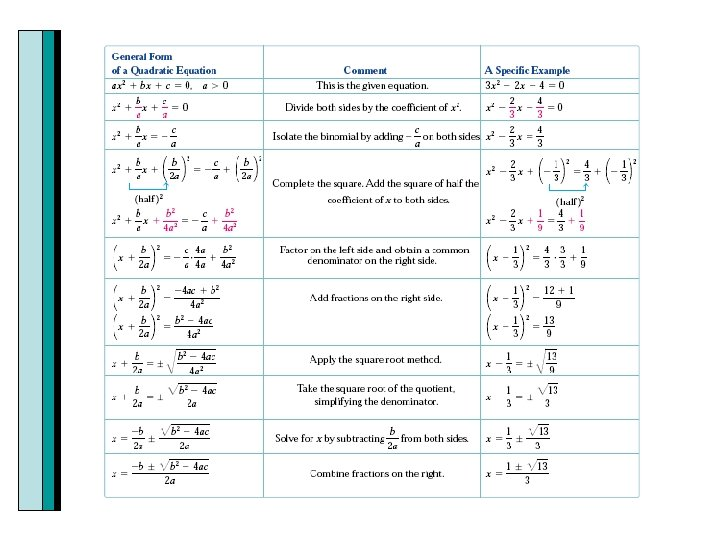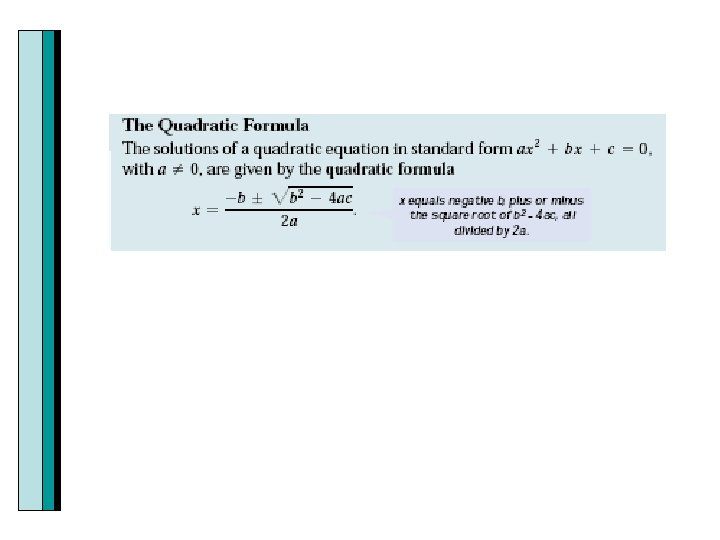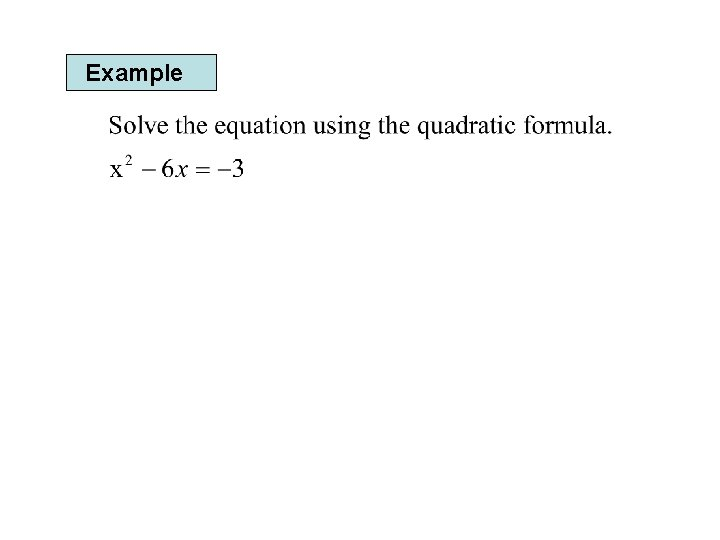Example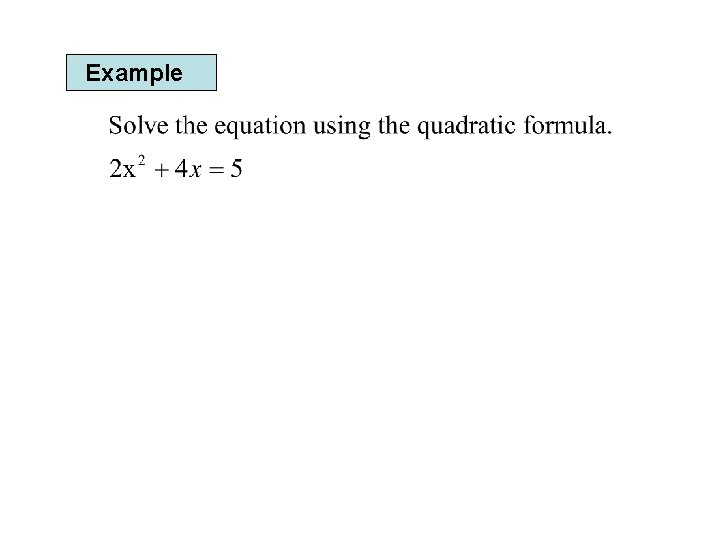Example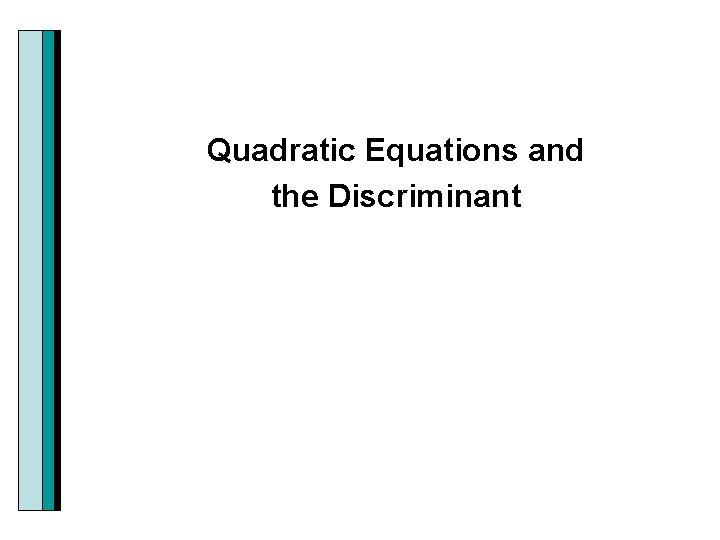ExampleGraphing Calculator The real solutions of a quadratic equation ax 2+bx+c=0 correspond to the x-intercepts of the graph. The U shaped graph shown below has two x intercepts. When y=0, the value(s) of x will be the solution to the equation. Since y=0 these are called the zeros of the function.Solving Polynomial Equations using the Graphing Calculator By pressing 2 nd Trace to get Calc, then the #2, you get the zeros. It will ask you for left and right bounds, and then a guess. For left and right bounds move the blinking cursor (using the arrow keys-cursor keys) to the left and press enter. Then move the cursor to the right of the x intercept and press enter. Press enter when asked to guess. Then you get the zeros or solution. Repeat this process for each x intercept.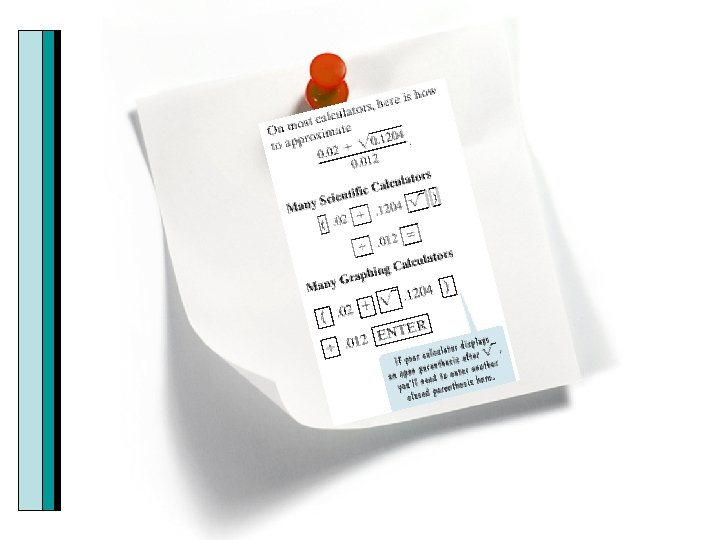Determining Which Method to Use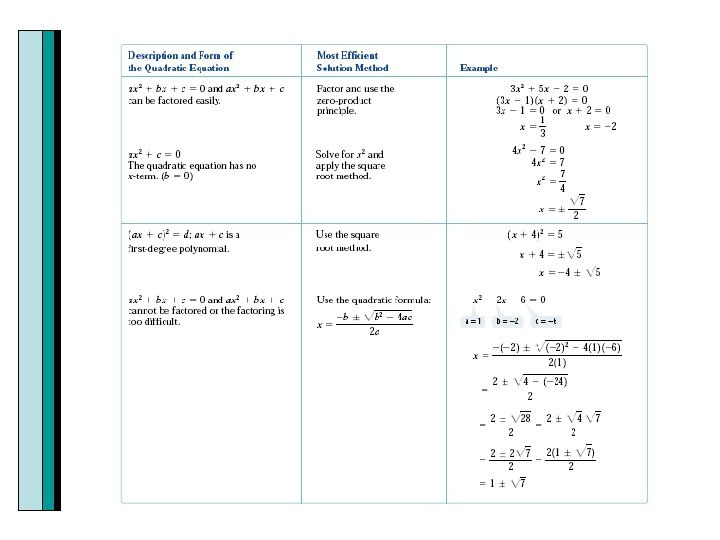Example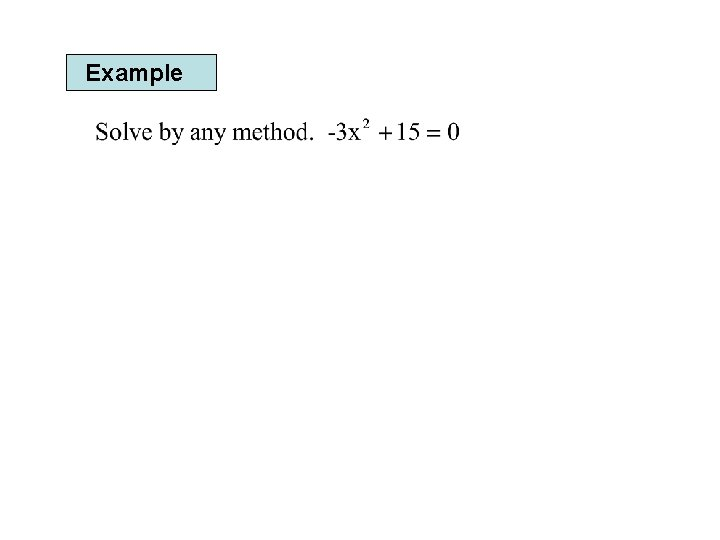Example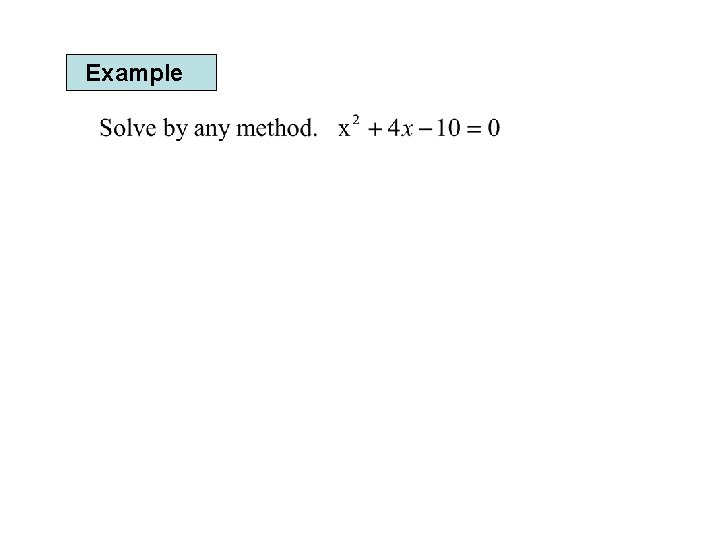Example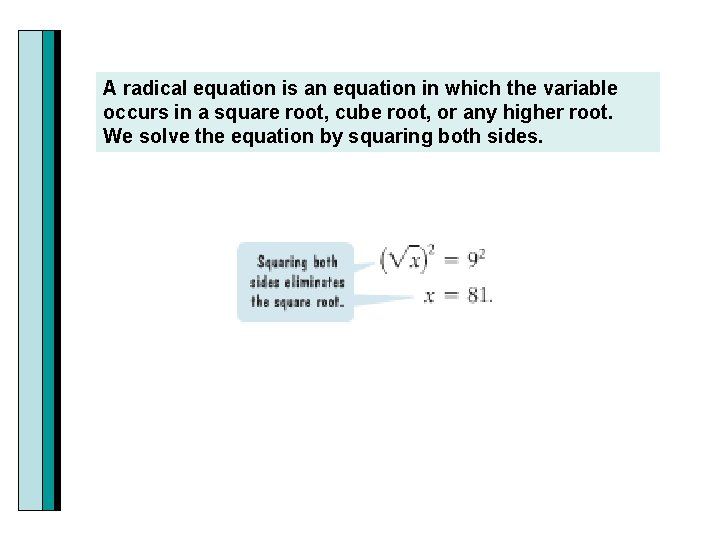A radical equation is an equation in which the variable occurs in a square root, cube root, or any higher root. We solve the equation by squaring both sides.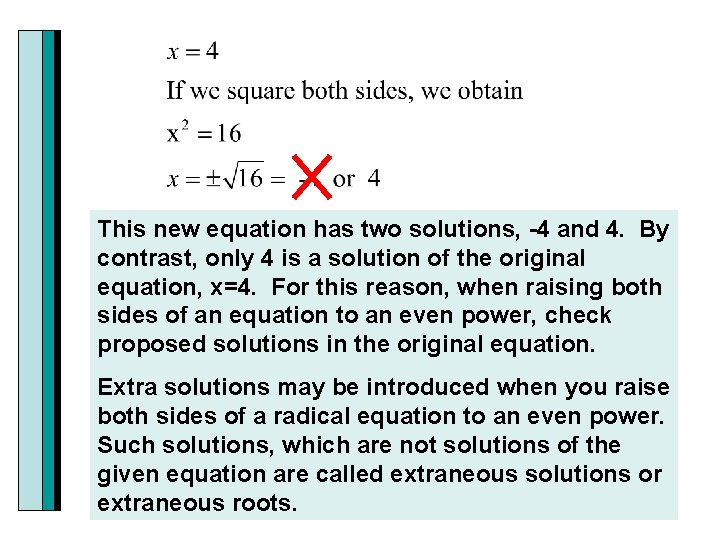This new equation has two solutions, -4 and 4. By contrast, only 4 is a solution of the original equation, x=4. For this reason, when raising both sides of an equation to an even power, check proposed solutions in the original equation. Extra solutions may be introduced when you raise both sides of a radical equation to an even power. Such solutions, which are not solutions of the given equation are called extraneous solutions or extraneous roots.